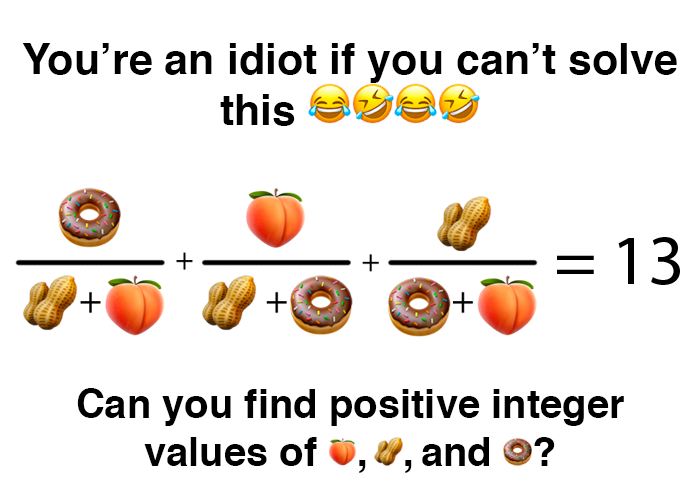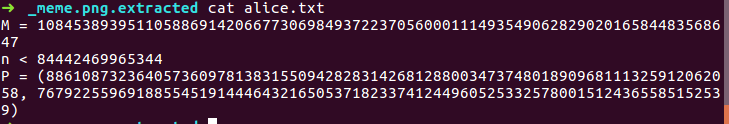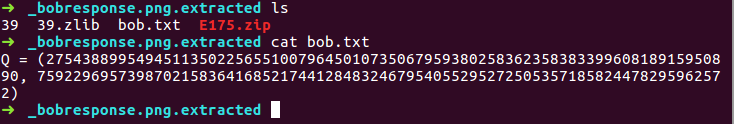# Alice sent Bob a meme - UTCTF 2019

tl;dr

1. Extract data from given images using binwalk
2. Tranform given diophantine equation into a cubic curve and retrieve EC parameters
3. Solve ECDLP given in extracted data using Pohlig Hellman Algorithm

Challenge Points: 1650
Challenge Solves: [Unknown]
Solved by: s0rc3r3r, v3ct0r & v4d3r

## Preliminary analysis

We are given three images: meme.png, screenshot.jpg and bobresponse.jpgIt basically contains a conversation between Alice and Bob in which they are sharing two images with each
other - meme.png and bobresponse.png.Transforming it into mathematical equation, we get the following:
$\frac{a}{b+c} + \frac{b}{a+c} + \frac{c}{a+b} = N = 13$

After attempting this problem on paper, we came to a conclusion that the problem given in this image is not
simple as it looks like.

Hence we tried searching solutions for this online and found this paper:

## Extracting data from PNG files

Next, we binwalk given images - meme.png and bobresponse.png to see for any files hidden inside the images:Hmm, looks like some challenge based on ECDLP. Okay, but how is it related to the diophantine equation we
discussed in the above section?

## Getting Elliptic Curve parameters

We find after reading this paper that homogenization of $\frac{a}{b+c} + \frac{b}{a+c} + \frac{c}{a+b} = N = 13$ is a cubic curve:

$y^2 = x^3 + (4*N^2 + 12*N - 3)*x^2 + 32*(N+3)*x$
$y^2 = x^3 + (4N^2 + 12N - 3)x^2 + 32(N+3)x$

For our challenge, N = 13. Hence,
$y^2 = x^3 + 829x^2 + 512x$

In our challenge, we also have one extra value in the file alice.txt extracted from meme.png: M. This made
us guess (probably?) that M is the modulus of the given curve.

We get the following curve: $y^2 \equiv x^3 + 829x^2 + 512x \mod M$

Let us move to solving the ECDLP!

## Solving ECDLP: PH Algorithm

P = (88610873236405736097813831550942828314268128800347374801890968111325912062058, 76792255969188554519144464321650537182337412449605253325780015124365585152539)

We also have value of $Q = x*P$, where * is scalar multiplication of a point P with k on an Elliptic Curve.

Q = (27543889954945113502256551007964501073506795938025836235838339960818915950890, 75922969573987021583641685217441284832467954055295272505357185824478295962572)

We also know one property about secret key x:
x < 84442469965344

We first checked if order of the base point P is factorisable, by using the following code:

We observe that order of the base point P is factorisable, making it favourable to Pohlig Hellman Algorithm:
[2^5 * 3^2 * 617 * 1031 * 460919 * 1284352459083875752760636625085191848403737033002118694776855821]

P also happens to be the generator of the curve, since order of subgroup generated by P is equal to
cardinality of the curve E

### Pohlig Hellman for solving ECDLP

Effectively reduce the time for DLP by solving DLP over factors of order of the base point of an Elliptic Curve!
$\_P\_order = p_1^{k_1}*p_2^{k_2}*p_3^{k_3}*…*p_n^{k_n}$

$Q = x*P$
Since, order of P is _P_order, value of Q will repeat after every _P_order values.

Similarly,
$Q*(\_P\_order/p_1^{k_1}) = x*(\_P\_order/p_1^{k_1})*P$
becomes discrete logarithm problem over mod p1k1. Hence, if p1k1 is small, we can easily solve DLP over
mod p1k1.

This can be generalised for all the factors p1k1, p2k2, …, pnkm to get:
$x\equiv a_1 \mod p_1^{k_1}$
$x\equiv a_2 \mod p_2^{k_2}$
$x\equiv a_3 \mod p_3^{k_3}$
$…$
$x\equiv a_n \mod p_n^{k_m}$

Now, we can apply CRT to get
$x\mod p_1^{k_1}*p_2^{k_2}*p_3^{k_3}*…*p_n^{k_m}$
$x\mod \_P\_order$

### Applying PH algo. to our challenge

As one can see, one of the factors of order of P is too large to be solved within a reasonable amount of time.
Here’s where the upper limit of secret value of x comes into place. We only need to solve DLP for factors such that their product is just greater than or equal to upper limit of x.

We implemented this in python/sage to get the secret key:

x = 1213123123131